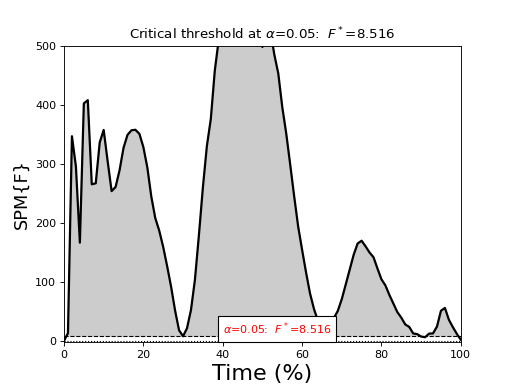# ANOVA¶

Note

p-values for ANOVA

In previous versions of spm1d no p values were generated for ANOVA because they had not yet been validated.

They have now been validated (Pataky, 2015) so now appear in results.

## One-way ANOVA¶

`./spm1d/examples/stats1d/ex_anova1.py`

Method 1 : stacked groups

If data for all groups is stored in a single (J x Q) array and A is a (J x 1) vector containing integers which specify the groups to which each observation belongs, then one-way ANOVA can be conducted like this:

```>>> F  = spm1d.stats.anova1( Y, A, equal_var=False )
>>> Fi = F.inference(alpha=0.05)
>>> Fi.plot()
```

Method 2 : separated groups

If there are K groups and data for each group are stored in separate (J_k x Q) variables, where J_k is the number of observations in the Kth group, then one-way ANOVA can be conducted like this:

```>>> F  = spm1d.stats.anova1( (Y1,Y2,Y3), equal_var=False )
>>> Fi = F.inference(alpha=0.05, interp=True)
>>> Fi.plot()
```## One-way repeated-measures ANOVA¶

`./spm1d/examples/stats1d/ex_anova1rm.py`

Repeated-measures ANOVA, also called “Within-subjects ANOVA”, can be conducted using Method 1 above and an additional (Jx1) vector of integers which specifies subjects.

```>>> F  = spm1d.stats.anova1rm( Y, A, SUBJ, equal_var=False )
>>> Fi = F.inference(alpha=0.05)
>>> Fi.plot()
```## Two-way ANOVA¶

Example:

```>>> FF  = spm1d.stats.anova2(Y, A, B, equal_var=False)
>>> FFi = [F.inference(alpha=0.05)   for F in FF]
>>> FFi.plot()   #Factor A main effect
>>> FFi.plot()   #Factor B main effect
>>> FFi.plot()   #Interaction effect
```

## Two-way nested ANOVA¶

Note

We do not yet have any real data examples to show.

The factor B is nested inside factor A.

```>>> F  = spm1d.stats.anova2nested( Y, A, B, equal_var=False )
```

## Two-way repeated-measures ANOVA¶

Note

We do not yet have any real data examples to show.

Implementing two-way repeated measures ANOVA requires a SUBJ vector:

```>>> F  = spm1d.stats.anova2rm( Y, A, B, SUBJ )
```

Danger

Non-sphericity corrections are not yet implemented for this design.

Since non-sphericity corrections weaken results with respect to assumed sphericity, interpret results cautiously, especially when close to alpha.

## Two-way ANOVA with repeated-measures on one factor¶

Note

We do not yet have any real data examples to show.

Implementing two-way repeated measures ANOVA requires a SUBJ vector.

B must be the repeated-measures factor.

```>>> F  = spm1d.stats.anova2onerm( Y, A, B, SUBJ )
```

Danger

Non-sphericity corrections are not yet implemented for this design.

Since non-sphericity corrections weaken results with respect to assumed sphericity, interpret results cautiously, especially when close to alpha.

## Three-way ANOVA¶

Note

We do not yet have any real data examples to show.

```>>> F  = spm1d.stats.anova3( Y, A, B, C, equal_var=False )
```

## Three-way nested ANOVA¶

Note

We do not yet have any real data examples to show.

This is a completely nested design: the factor C is nested inside factor B, which is in turn nested inside factor A.

spm1d currently does not support partial nesting.

```>>> F  = spm1d.stats.anova3nested( Y, A, B, C, equal_var=False )
```

## Three-way ANOVA with repeated-measures on two factors¶

Note

We do not yet have any real data examples to show.

Implementing three-way repeated measures ANOVA requires a SUBJ vector.

B and C must be the repeated-measures factor.

```>>> F  = spm1d.stats.anova3tworm( Y, A, B, C, SUBJ )
```

Danger

Non-sphericity corrections are not yet implemented for this design.

Since non-sphericity corrections weaken results with respect to assumed sphericity, interpret results cautiously, especially when close to alpha.

## Three-way ANOVA with repeated-measures on one factor¶

Note

We do not yet have any real data examples to show.

Implementing three-way repeated measures ANOVA requires a SUBJ vector.

B and C must be the repeated-measures factor.

```>>> F  = spm1d.stats.anova3onerm( Y, A, B, C, SUBJ )
```

Danger

Non-sphericity corrections are not yet implemented for this design.

Since non-sphericity corrections weaken results with respect to assumed sphericity, interpret results cautiously, especially when close to alpha.